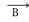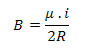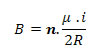Modern Physics

# Field in a circular loop

Circular loop carried by an electric current supplied by the battery

It assumes that a straight wire carries a direct current. Also consider that this same wire is bent to form a flat circular loop of radius R, carried by an electric current of intensity i, as shown in the figure above.

In a flat circular loop, the magnetic field lines are circles perpendicular to its plane, concentric with the conductor. The magnetic induction vectorat the center O of this loop has the following characteristics:

– intensity : the intensity of the vectorat the center of the loop is given by the expression:– direction : normal to the plane of the loop;
– sense : given by the right hand rule.

Through the right hand rule we can see that vector B is vertical.

The induction lines of a magnet leave the north pole and arrive at the south pole. A loop carried by an electric current creates a magnetic field similar to that of the magnet and, then, a north pole is assigned to it, from which the lines come out; and a south pole, to which they arrive.

If we consider n equal turns juxtaposed, that is, one next to the other, so that the thickness of the winding is much smaller than the diameter of each turn, we will have the so-called flat coil . The intensity of the magnetic induction vector B at the center O of the flat coil is determined by the following equation:Where:

B – is the magnetic field inside the loop (or coil)
i – is the electric current
R – is the radius of the loop (or coil)
μ – is the magnetic permeability
n – is the number of turns of the coil